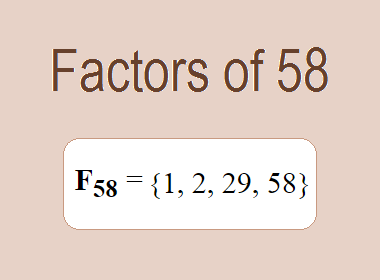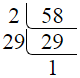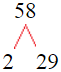# Factors of 58The factors of 58 are 1, 2, 29, and 58 i.e. F58 = {1, 2, 29, 58}. The factors of 58 are all the numbers that can divide 58 without leaving a remainder.

We can check if these numbers are factors of 58 by dividing 58 by each of them. If the result is a whole number, then the number is a factor of 58. Let's do this for each of the numbers listed above:

·        1 is a factor of 58 because 58 divided by 1 is 58.

·        2 is a factor of 58 because 58 divided by 2 is 29.

·        29 is a factor of 58 because 58 divided by 29 is 2.

·        58 is a factor of 58 because 58 divided by 58 is 1.

## How to Find Factors of 58?

1 and the number itself are the factors of every number. So, 1 and 58 are two factors of 58. To find the other factors of 58, we can start by dividing 58 by the numbers between 1 and 58. If we divide 58 by 2, we get a remainder of 0. Therefore, 2 is a factor of 58. If we divide 58 by 3, we get a remainder of 1. Therefore, 3 is not a factor of 58.

Next, we can check if 4 is a factor of 58. If we divide 58 by 4, we get a remainder of 2. Therefore, 4 is not a factor of 58. We can continue this process for all the possible factors of 58.

Through this process, we can find that the factors of 58 are 1, 2, 29, and 58. These are the only numbers that can divide 58 without leaving a remainder.

********************

********************

## Properties of the Factors of 58

The factors of 58 have some interesting properties. One of the properties is that the sum of the factors of 58 is equal to 90. We can see this by adding all the factors of 58 together:

1 + 2 + 29 + 58 = 90

Another property of the factors of 58 is that the prime factors of 58 are 2 and 29.

## Applications of the Factors of 58

The factors of 58 have several applications in mathematics. One of the applications is in finding the highest common factor (HCF) of two or more numbers. The HCF is the largest factor that two or more numbers have in common. For example, to find the HCF of 58 and 29, we need to find the factors of both numbers and identify the largest factor they have in common. The factors of 58 are 1, 2, 29, and 58. The factors of 29 are 1, and 29. The largest factor that they have in common is 29. Therefore, the HCF of 58 and 29 is 29.

Another application of the factors of 58 is in prime factorization. Prime factorization is the process of expressing a number as the product of its prime factors. The prime factors of 58 are 2 and 29 since these are the only prime numbers that can divide 58 without leaving a remainder. Therefore, we can express 58 as:

58 = 2 × 29

We can do prime factorization by division and factor tree method also. Here is the prime factorization of 58 by division method,58 = 2 × 29

Here is the prime factorization of 58 by the factor tree method,58 = 2 × 29

## Conclusion

The factors of 58 are the numbers that can divide 58 without leaving a remainder. The factors of 58 are 1, 2, 29, and 58. The factors of 58 have some interesting properties, such as having a sum of 90. The factors of 58 have several applications in mathematics, such as finding the highest common factor and prime factorization.